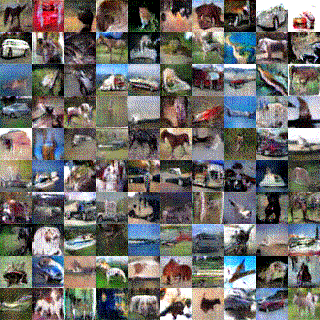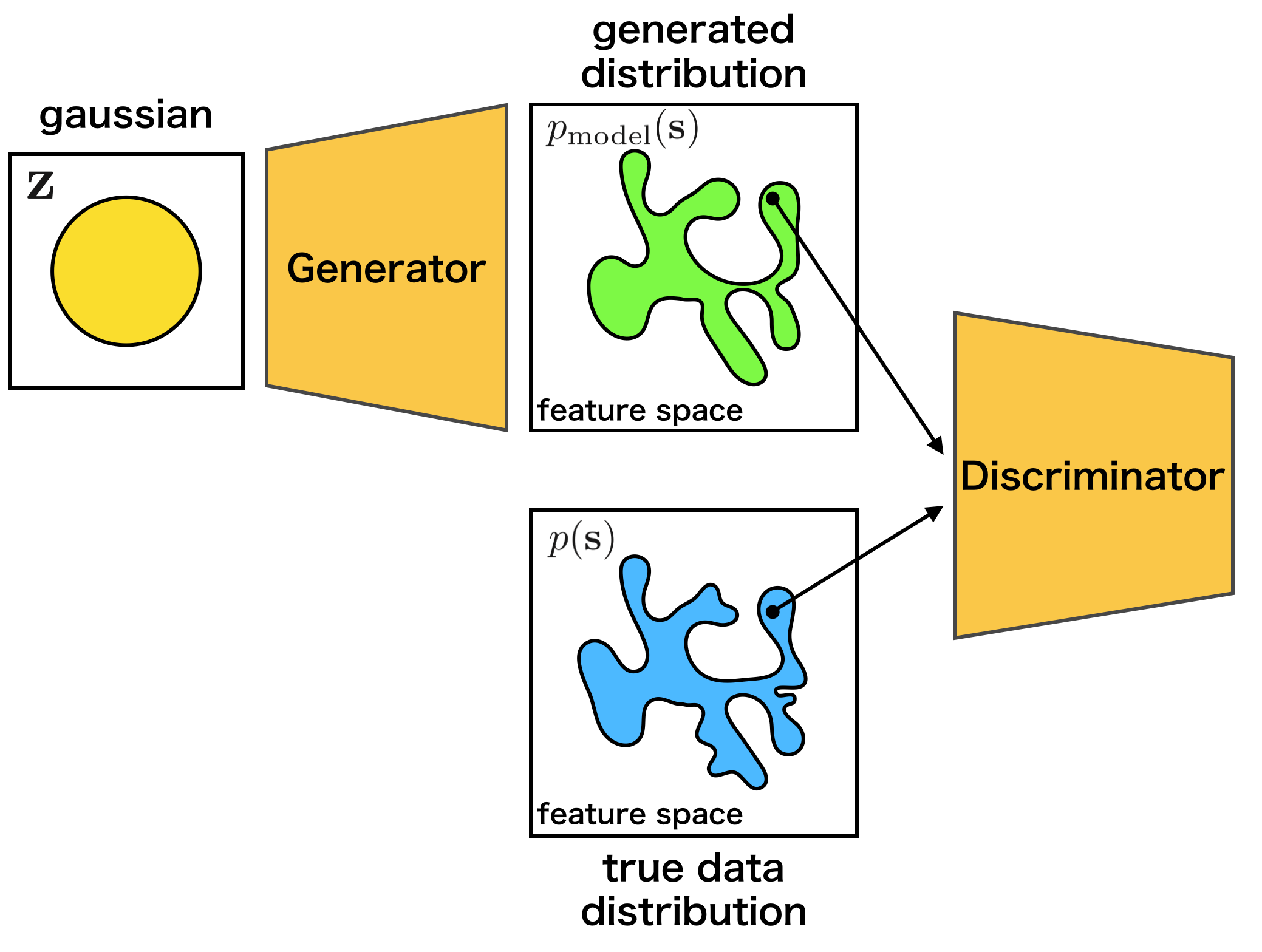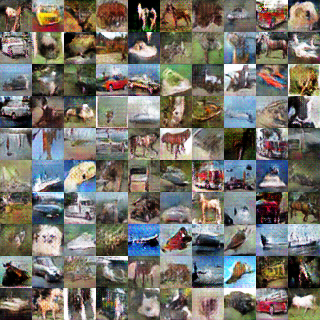# DCGAN: Generate images with Deep Convolutional GAN¶

## 0. Introduction¶

In this tutorial, we generate images with generative adversarial networks (GAN). GAN are kinds of deep neural network for generative modeling that are often applied to image generation. GAN-based models are also used in PaintsChainer, an automatic colorization service.In this tutorial, you will learn the following things:

1. Generative Adversarial Networks (GAN)

2. Implementation of DCGAN in Chainer

## 1. Generarive Adversarial Networks (GAN)¶

### 1.1 What are GAN?¶

As explained in GAN tutorial in NIPS 2016 , generative models can be classified into the categories as shown in the following figure:

Besides GAN, other famous generative models include Fully visible belief networks (FVBNs) and Variational autoencoder (VAE). Unlike FVBNs and VAE, GAN do not explicitly model the probability distribution $$p({\bf s})$$ that generates training data. Instead, we model a generator $$G: {\bf z} \mapsto {\bf s}$$. The generator $$G$$ samples $${\bf s} \sim p({\bf s})$$ from the latent variable $${\bf z}$$. Apart from the generator $$G$$, we create a discriminator $$D({\bf x})$$ which discriminates between samples from the generator G and examples from training data. While training the discriminator $$D$$, the generator $$G$$ tries to maximize the probability of the discriminator $$D$$ making a mistake. So, the generator $$G$$ tries to create samples that seem to be drawn from the same distribution as the training data.

The advantages of GAN are low sampling cost and its state-of-the-art performance in image generation. The disadvantage is that we cannot calculate the likelihood $$p_{\mathrm {model}}({\bf s})$$ because we do not model any probability distribution, and we cannot infer the latent variable $${\bf z}$$ from a sample.

### 1.2 How GAN work?¶

As explained above, GAN use the two models, the generator and the discriminator. When training the networks, we should match the data distribution $$p({\bf s})$$ with the distribution of the samples $${\bf s} = G ({\bf z})$$ generated from the generator.The generator $$G$$ learns the target distribution, and ideally eventually reaches a Nash equilibrium  of game theory. In detail, while training the discriminator $$D$$, the generator $$G$$ is also trained, so that the discriminator $$D$$ makes a mistake.

As an intuitive example, the relationship between counterfeiters of banknotes and the police is frequently used. The counterfeiters try to make counterfeit notes that look like real banknotes. The police try to distinguish real bank notes from counterfeit notes. It is supposed that the ability of the police gradually rises, so that real banknotes and counterfeit notes can be recognized well. Then, the counterfeiters will not be able to use counterfeit banknotes, so they will create counterfeit banknotes that appear more realistic. As the police improve their skill further, they can distinguish real and counterfeit notes… Eventually, the counterfeiter will be able to produce counterfeit banknotes look as real as genuine ones.

The training process is explained by the following mathematical expressions. First, since the discriminator $$D({\bf s})$$ is the probability that a sample $${\bf s}$$ is generated from the data distribution at, it can be expressed as follows:

$D({\bf s}) = \frac{p({\bf s})}{p({\bf s}) + p_{\mathrm{model}}({\bf s})}$

Then, when we match the data distribution $${\bf s} \sim p({\bf s})$$ and the distribution of generated samples by $$G$$, it means that we should minimize the dissimilarity between the two distributions. It is common to use Jensen-Shannon Divergence $$D_{\mathrm{JS}}$$ to measure the dissimilarity between distributions.

The $$D_{\mathrm{JS}}$$ of $$p_{\mathrm{model}}({\bf s})$$ and $$p({\bf s})$$ can be written as follows by using $$D({\bf s})$$:

$\begin{split}2 D_{\mathrm{JS}} &=& D_{\mathrm{KL}}(p({\bf s})||\bar{p}({\bf s})) + D_{\mathrm{KL}}(p_{\mathrm{model}}({\bf s})||\bar{p}({\bf s})) \\ &=& \mathbb{E}_{p({\bf s})} \left[ \log \frac{2p({\bf s})}{p({\bf s}) + p_{\mathrm{model}}({\bf s})} \right] + \mathbb{E}_{p_{\mathrm{model}}} \left[ \log \frac{2p_{\mathrm{model}}({\bf s})}{p({\bf s}) + p_{\mathrm{model}}({\bf s})} \right] \\ &=& \mathbb{E}_{p({\bf s})} \log D({\bf s}) + \mathbb{E}_{p_{\mathrm{model}}} \log (1-D({\bf s})) + \log 4 \\ &=& \mathbb{E}_{p({\bf s})} \log D({\bf s}) + \mathbb{E}_{p_{\bf z}} \log (1-D(G({\bf z}))) + \log 4\end{split}$

where $$\bar{p}({\bf s}) = \frac{p({\bf s}) + p_{\rm model}({\bf s})}{2}$$. The $$D_{\mathrm{JS}}$$ will be maximized by the discriminator $$D$$ and minimized by the generator $$G$$, namely, $$p_{\mathrm{model}}$$. And the distribution $$p_{\mathrm model}({\bf s})$$ generated by $$G({\bf {\bf s}})$$ can match the data distribution $$p({\bf s})$$.

$\min_{G} \max_{D} \mathbb{E}_{p({\bf s})} \log D({\bf s}) + \mathbb{E}_{p_{\bf z}} \log (1-D(G({\bf z})))$

When we actually train the model, the above min-max problem is solved by alternately updating the discriminator $$D({\bf s})$$ and the generator $$G({\bf z})$$ . The actual training procedures are described as follows:

### 1.3 What are DCGAN?¶

In this section, we will introduce the model called DCGAN(Deep Convolutional GAN) proposed by Radford et al.. As shown below, it is a model using CNN(Convolutional Neural Network) as its name suggests.

In addition, although GAN are known for its difficulty in training, this paper introduces various techniques for successful training:

1. Convert max-pooling layers to convolution layers with larger or fractional strides

2. Convert fully connected layers to global average pooling layers in the discriminator

3. Use batch normalization layers in the generator and the discriminator

4. Use leaky ReLU activation functions in the discriminator

## 2. Implementation of DCGAN in Chainer¶

There is an example of DCGAN in the official repository of Chainer, so we will explain how to implement DCGAN based on this: chainer/examples/dcgan

### 2.1 Define the generator model¶

First, let’s define a network for the generator.

train_dcgan.py
class Generator(chainer.Chain):

def __init__(self, n_hidden, bottom_width=4, ch=512, wscale=0.02):
super(Generator, self).__init__()
self.n_hidden = n_hidden
self.ch = ch
self.bottom_width = bottom_width

with self.init_scope():
w = chainer.initializers.Normal(wscale)
self.l0 = L.Linear(self.n_hidden, bottom_width * bottom_width * ch,
initialW=w)
self.dc1 = L.Deconvolution2D(ch, ch // 2, 4, 2, 1, initialW=w)
self.dc2 = L.Deconvolution2D(ch // 2, ch // 4, 4, 2, 1, initialW=w)
self.dc3 = L.Deconvolution2D(ch // 4, ch // 8, 4, 2, 1, initialW=w)
self.dc4 = L.Deconvolution2D(ch // 8, 3, 3, 1, 1, initialW=w)
self.bn0 = L.BatchNormalization(bottom_width * bottom_width * ch)
self.bn1 = L.BatchNormalization(ch // 2)
self.bn2 = L.BatchNormalization(ch // 4)
self.bn3 = L.BatchNormalization(ch // 8)

def make_hidden(self, batchsize):
dtype = chainer.get_dtype()
return numpy.random.uniform(-1, 1, (batchsize, self.n_hidden, 1, 1))\
.astype(dtype)

def forward(self, z):
h = F.reshape(F.relu(self.bn0(self.l0(z))),
(len(z), self.ch, self.bottom_width, self.bottom_width))
h = F.relu(self.bn1(self.dc1(h)))
h = F.relu(self.bn2(self.dc2(h)))
h = F.relu(self.bn3(self.dc3(h)))
x = F.sigmoid(self.dc4(h))
return x


When we make a network in Chainer, there are some conventions:

1. Define a network class which inherits Chain.

2. Make chainer.links‘s instances in the init_scope(): of the initializer __init__.

3. Define network connections in the __call__ operator by using the chainer.links‘s instances and chainer.functions.

If you are not familiar with constructing a new network, please refer to this tutorial.

As we can see from the initializer __init__, the Generator uses deconvolution layers Deconvolution2D and batch normalization layers BatchNormalization. In __call__, each layer is called and followed by relu except the last layer.

Because the first argument of L.Deconvolution is the channel size of input and the second is the channel size of output, we can find that each layer halves the channel size. When we construct Generator with ch=1024, the network is same as the above image.

Note

Be careful when passing the output of a fully connected layer to a convolution layer, because the convolutional layer needs additional dimensions for inputs. As we can see the 1st line of __call__, the output of the fully connected layer is reshaped by reshape to add the dimensions of the channel, the width and the height of images.

### 2.2 Define the discriminator model¶

In addition, let’s define the network for the discriminator.

train_dcgan.py
class Discriminator(chainer.Chain):

def __init__(self, bottom_width=4, ch=512, wscale=0.02):
w = chainer.initializers.Normal(wscale)
super(Discriminator, self).__init__()
with self.init_scope():
self.c0_0 = L.Convolution2D(3, ch // 8, 3, 1, 1, initialW=w)
self.c0_1 = L.Convolution2D(ch // 8, ch // 4, 4, 2, 1, initialW=w)
self.c1_0 = L.Convolution2D(ch // 4, ch // 4, 3, 1, 1, initialW=w)
self.c1_1 = L.Convolution2D(ch // 4, ch // 2, 4, 2, 1, initialW=w)
self.c2_0 = L.Convolution2D(ch // 2, ch // 2, 3, 1, 1, initialW=w)
self.c2_1 = L.Convolution2D(ch // 2, ch // 1, 4, 2, 1, initialW=w)
self.c3_0 = L.Convolution2D(ch // 1, ch // 1, 3, 1, 1, initialW=w)
self.l4 = L.Linear(bottom_width * bottom_width * ch, 1, initialW=w)
self.bn0_1 = L.BatchNormalization(ch // 4, use_gamma=False)
self.bn1_0 = L.BatchNormalization(ch // 4, use_gamma=False)
self.bn1_1 = L.BatchNormalization(ch // 2, use_gamma=False)
self.bn2_0 = L.BatchNormalization(ch // 2, use_gamma=False)
self.bn2_1 = L.BatchNormalization(ch // 1, use_gamma=False)
self.bn3_0 = L.BatchNormalization(ch // 1, use_gamma=False)

def forward(self, x):
device = self.device
h = add_noise(device, x)
h = F.leaky_relu(add_noise(device, self.c0_0(h)))
h = F.leaky_relu(add_noise(device, self.bn0_1(self.c0_1(h))))
h = F.leaky_relu(add_noise(device, self.bn1_0(self.c1_0(h))))
h = F.leaky_relu(add_noise(device, self.bn1_1(self.c1_1(h))))
h = F.leaky_relu(add_noise(device, self.bn2_0(self.c2_0(h))))
h = F.leaky_relu(add_noise(device, self.bn2_1(self.c2_1(h))))
h = F.leaky_relu(add_noise(device, self.bn3_0(self.c3_0(h))))
return self.l4(h)


The Discriminator network is almost mirrors of the Generator network. However, there are minor different points:

1. Use leaky_relu as activation functions

2. Deeper than Generator

3. Add some noise to every intermediate outputs before giving them to the next layers

train_dcgan.py
def add_noise(device, h, sigma=0.2):
if chainer.config.train:
xp = device.xp
# TODO(niboshi): Support random.randn in ChainerX
if device.xp is chainerx:
fallback_device = device.fallback_device
with chainer.using_device(fallback_device):
randn = device.send(fallback_device.xp.random.randn(*h.shape))
else:
randn = xp.random.randn(*h.shape)
return h + sigma * randn
else:
return h


### 2.3 Prepare dataset and iterator¶

Let’s retrieve the CIFAR-10 dataset by using Chainer’s dataset utility function get_cifar10. CIFAR-10 is a set of small natural images. Each example is an RGB color image of size 32x32. In the original images, each of R, G, B of pixels is represented by one-byte unsigned integer (i.e. from 0 to 255). This function changes the scale of pixel values into [0, scale] float values.

    train, _ = chainer.datasets.get_cifar10(withlabel=False, scale=255.)

train_dcgan.py
train_iter = chainer.iterators.SerialIterator(train, args.batchsize)



### 2.4 Prepare model and optimizer¶

Let’s make the instances of the generator and the discriminator.

train_dcgan.py
gen = Generator(n_hidden=args.n_hidden)
dis = Discriminator()

gen.to_device(device)  # Copy the model to the device
dis.to_device(device)

# Setup an optimizer
def make_optimizer(model, alpha=0.0002, beta1=0.5):
optimizer = chainer.optimizers.Adam(alpha=alpha, beta1=beta1)
optimizer.setup(model)
chainer.optimizer_hooks.WeightDecay(0.0001), 'hook_dec')
return optimizer

opt_gen = make_optimizer(gen)
opt_dis = make_optimizer(dis)



Next, let’s make optimizers for the models created above.

train_dcgan.py
def make_optimizer(model, alpha=0.0002, beta1=0.5):
optimizer = chainer.optimizers.Adam(alpha=alpha, beta1=beta1)
optimizer.setup(model)
chainer.optimizer_hooks.WeightDecay(0.0001), 'hook_dec')
return optimizer

opt_gen = make_optimizer(gen)
opt_dis = make_optimizer(dis)



### 2.5 Prepare updater¶

GAN need the two models: the generator and the discriminator. Usually, the default updaters pre-defined in Chainer take only one model. So, we need to define a custom updater for GAN training.

The definition of DCGANUpdater is a little complicated. However, it just minimizes the loss of the discriminator and that of the generator alternately.

As you can see in the class definition, DCGANUpdater inherits StandardUpdater. In this case, almost all necessary functions are defined in StandardUpdater, we just override the functions of __init__ and update_core.

Note

We do not need to define loss_dis and loss_gen because the functions are called only in update_core. It aims at improving readability.

train_dcgan.py
class DCGANUpdater(chainer.training.updaters.StandardUpdater):

def __init__(self, *args, **kwargs):
self.gen, self.dis = kwargs.pop('models')
super(DCGANUpdater, self).__init__(*args, **kwargs)

def loss_dis(self, dis, y_fake, y_real):
batchsize = len(y_fake)
L1 = F.sum(F.softplus(-y_real)) / batchsize
L2 = F.sum(F.softplus(y_fake)) / batchsize
loss = L1 + L2
chainer.report({'loss': loss}, dis)
return loss

def loss_gen(self, gen, y_fake):
batchsize = len(y_fake)
loss = F.sum(F.softplus(-y_fake)) / batchsize
chainer.report({'loss': loss}, gen)
return loss

def update_core(self):
gen_optimizer = self.get_optimizer('gen')
dis_optimizer = self.get_optimizer('dis')

batch = self.get_iterator('main').next()
device = self.device
x_real = Variable(self.converter(batch, device)) / 255.

gen, dis = self.gen, self.dis
batchsize = len(batch)

y_real = dis(x_real)

z = Variable(device.xp.asarray(gen.make_hidden(batchsize)))
x_fake = gen(z)
y_fake = dis(x_fake)

dis_optimizer.update(self.loss_dis, dis, y_fake, y_real)
gen_optimizer.update(self.loss_gen, gen, y_fake)


In the initializer __init__, an additional keyword argument models is required as you can see the code below. Also, we use keyword arguments iterator, optimizer and device. It should be noted that the optimizer augment takes a dictionary. The two different models require two different optimizers. To specify the different optimizers for the models, we give a dictionary, {'gen': opt_gen, 'dis': opt_dis}, to the optimizer argument. we should input optimizer as a dictionary {'gen': opt_gen, 'dis': opt_dis}. In the DCGANUpdater, you can access the iterator with self.get_iterator('main'). Also, you can access the optimizers with self.get_optimizer('gen') and self.get_optimizer('dis').

In update_core, the two loss functions loss_dis and loss_gen are minimized by the optimizers. At first two lines, we access the optimizers. Then, we create next minibatch of training data by self.get_iterator('main').next(), copy batch to the device by self.converter, and make it a Variable object. After that, we minimize the loss functions with the optimizers.

Note

When defining update_core, we may want to manipulate the underlying array of a Variable with numpy or cupy library. Note that the type of arrays on CPU is numpy.ndarray, while the type of arrays on GPU is cupy.ndarray. However, users do not need to write if condition explicitly, because the appropriate array module can be obtained by xp = chainer.backend.get_array_module(variable.array). If variable is on GPU, cupy is assigned to xp, otherwise numpy is assigned to xp.

train_dcgan.py
updater = DCGANUpdater(
models=(gen, dis),
iterator=train_iter,
optimizer={
'gen': opt_gen, 'dis': opt_dis},
device=device)



### 2.6 Prepare trainer and run¶

train_dcgan.py
trainer = training.Trainer(updater, (args.epoch, 'epoch'), out=args.out)

snapshot_interval = (args.snapshot_interval, 'iteration')
display_interval = (args.display_interval, 'iteration')
trainer.extend(
extensions.snapshot(filename='snapshot_iter_{.updater.iteration}.npz'),
trigger=snapshot_interval)
trainer.extend(extensions.snapshot_object(
gen, 'gen_iter_{.updater.iteration}.npz'), trigger=snapshot_interval)
trainer.extend(extensions.snapshot_object(
dis, 'dis_iter_{.updater.iteration}.npz'), trigger=snapshot_interval)
trainer.extend(extensions.LogReport(trigger=display_interval))
trainer.extend(extensions.PrintReport([
'epoch', 'iteration', 'gen/loss', 'dis/loss',
]), trigger=display_interval)
trainer.extend(extensions.ProgressBar(update_interval=10))
trainer.extend(
out_generated_image(
gen, dis,
10, 10, args.seed, args.out),
trigger=snapshot_interval)


train_dcgan.py
trainer.run()



### 2.7 Start training¶

We can run the example as follows.

$pwd /root2chainer/chainer/examples/dcgan$ python train_dcgan.py --gpu 0
GPU: 0
# Minibatch-size: 50
# n_hidden: 100
# epoch: 1000

epoch       iteration   gen/loss    dis/loss  ................]  0.01%
0           100         1.2292      1.76914
total [..................................................]  0.02%
this epoch [#########.........................................] 19.00%
190 iter, 0 epoch / 1000 epochs
10.121 iters/sec. Estimated time to finish: 1 day, 3:26:26.372445.


The results will be saved in the directory /root2chainer/chainer/examples/dcgan/result/. The image is generated by the generator trained for 1000 epochs, and the GIF image on the top of this page shows generated images after every 10 epochs.